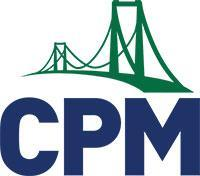# Mathematics Program

Eureka/EngageNY Math

The K-8 students of Uinta County School District #1 use the Eureka Math from GreatMinds Math Curriculum.  Eureka Math, also known as Engage NY, is a math curriculum that sequences the mathematical progressions in a logical order in modules.  This curriculum is designed to reduce gaps in student learning, instill persistence in problem solving, and prepare students to understand advanced math.

College Preparatory Mathematics (CPM)College Preparatory Mathematics (CPM0 is comprised of a five-year sequence of college preparatory mathematics courses that starts with Core Connections Integrated I and continues through Calculus Third Edition. It aims to deepen and extend student understanding built in previous courses by focusing on developing fluency with solving linear equations, inequalities, and systems. These skills are extended to solving simple exponential equations, exploring linear and exponential functions graphically, numerically, symbolically, and as sequences, and by using regression techniques to analyze the fit of models to distributions of data.

On a daily basis, students use problem-solving strategies, questioning, investigating, analyzing critically, gathering and constructing evidence, and communicating rigorous arguments justifying their thinking. Under teacher guidance, students lean in collaboration with others while sharing information, expertise, and ideas. The program offers a well balanced approach among procedural fluency (algorithms and basic skills), deep conceptual understanding, strategic competence (problem solving), and adaptive reasoning (extension and application). The courses embed the CCSS Standards for Mathematical Practices as an integral part of the lessons in the course.

Math Curriculum & Resources